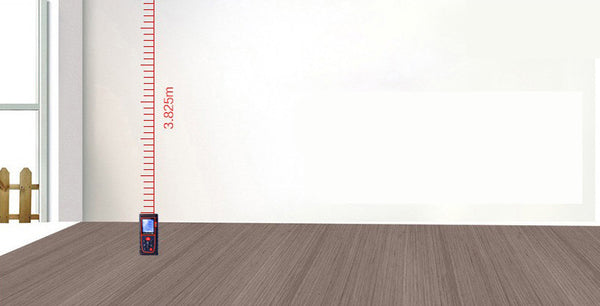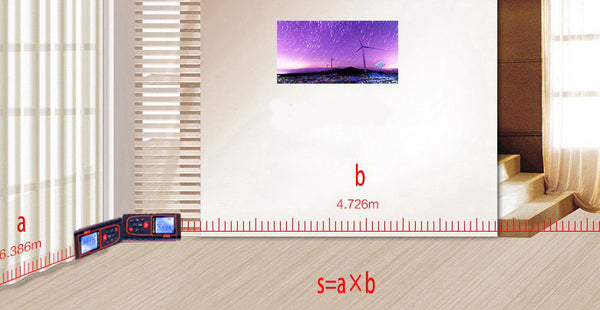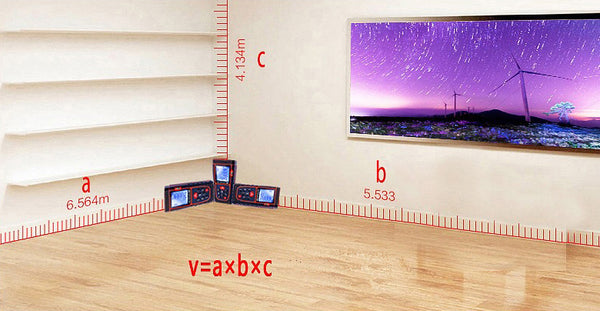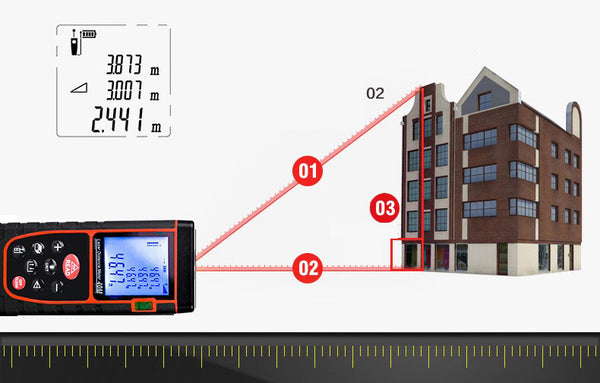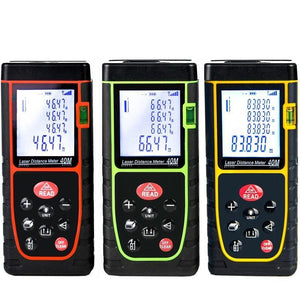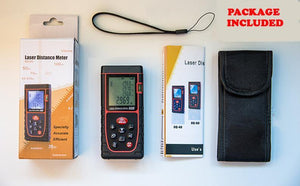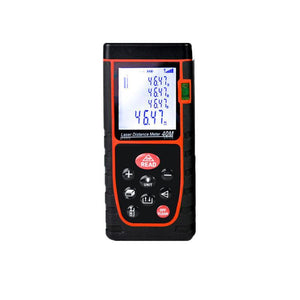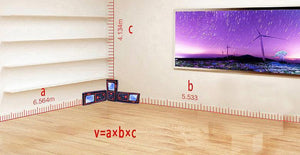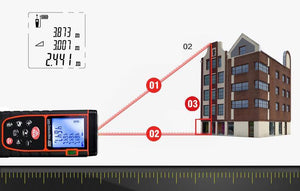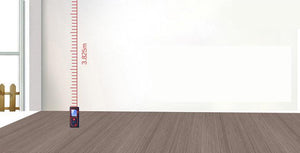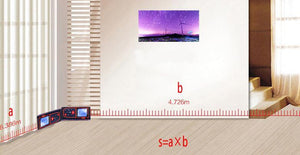# Laser Distance Meter

Regular price
\$39.99
Unit price  per302 watching 99 sold in the last hour
179 of 224 sold

Small doesn't mean modest in a laser measure with functions that include area, distance, length, volume, continuous measurement, and addition/subtraction. Simply press the measure button to activate the laser and begin measuring in real time. Backlighting allows user to see information in dark areas with better resolution. Handy pocket-size design makes unit easy to use anywhere. The Laser Distance Meter measures to 130feet.

Use method
1. Place the rangefinder over the wall, or place it on the floor, or simply hold it on your hand.
2. Press the distance button to turn on the laser.
3. Point the laser at the target, press the distance button again, and read the measured reading directly from the screen.
4. When measuring the area, press the area button and then measure the length and width. The Laser Distance Meter will automatically calculate the area.
5. While measuring the volume, press the Volume button and measure the length, width, and height.
To add or subtract the distance, first measure a distance, and then press the plus or minus sign to add or subtract the next distance. Use this function to measure the perimeter, non-linear distance (add multiple short distances), and measure directly (measure the entire segment and subtract a small segment to get the remaining distance)

Widely used in a high degree of measurement, area measurement, volume measurement, hook shares measurement; 20 sets of data storage.